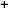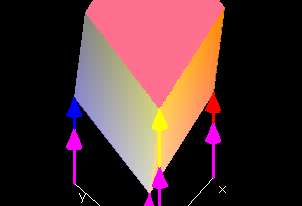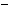Labware - MA35 Multivariable Calculus - Three Variable Calculus
 MA35 Labs 3 » Three Variable Calculus Contents3.1 Functions of Three Variables 3.1.1 Linear Functions 3.1.5 Continuity 3.4 Integration Search

Linear Functions

Text

Calculus is the study of functions.Demos

 Linear FunctionsThis demo shows the linear function in three variables L(x,y,z) = px + qy +k over the rectangular domain 0 ≤ x ≤ 1, 0 ≤ y ≤ 1, 0 ≤ z ≤ 1. You can change the constants p,q, r and k using the respective red, blue, yellow or magenta slide bar, and the Graph: L(x,y,z) window will show the patch of the function graph of L(x,y,z) with the chosen values for the constants over the rectangular domain. Note, however, that the function graph is not displayed entirely correctly. In three-dimensional space, it is not possible to display the four mutually perpendicular axeed in the directions of x,y,z and f(x,y,z). Therefore, the fourth axis in f(x,y,z)-direction (indicated by the magenta arrows) is not perpendicular to any of the other directions. If you slide the hotspot on the purple slidebar, (thereby varying the constant k) the function graph is translated up and down in the directon of f(x,y,z). Varying p,q and r on the red, blue and yellow slidebars will scale in the x-,y- and z-direction of the graph. Note: For help on using demos, see page 1 of lab 2.1.1.Examples

The Zero FunctionConstant FunctionsLinear FunctionsExercises

• 1. Show that if p ≠ 0, then for every w there is a point (x,y,z) such that L(x,y,z) = px + qy + rz + k = w. Show that if q ≠ 0 or r ≠ 0, then the same result holds.
• 2. Consider the tetrahedron with vertices at the x-intercept, y-intercept, z-intercept, and w-intercept (i.e. x = y = z = 0) points of the graph of L(x,y,z) = px + qy + rz + k. For what values of p, q, r, and k does this tetrahedron intersect the graph of L(x,y,z) over the domain 0 {leq} x {leq} 1, 0 {leq} y {leq} 1, 0 {leq} z {leq} 1?